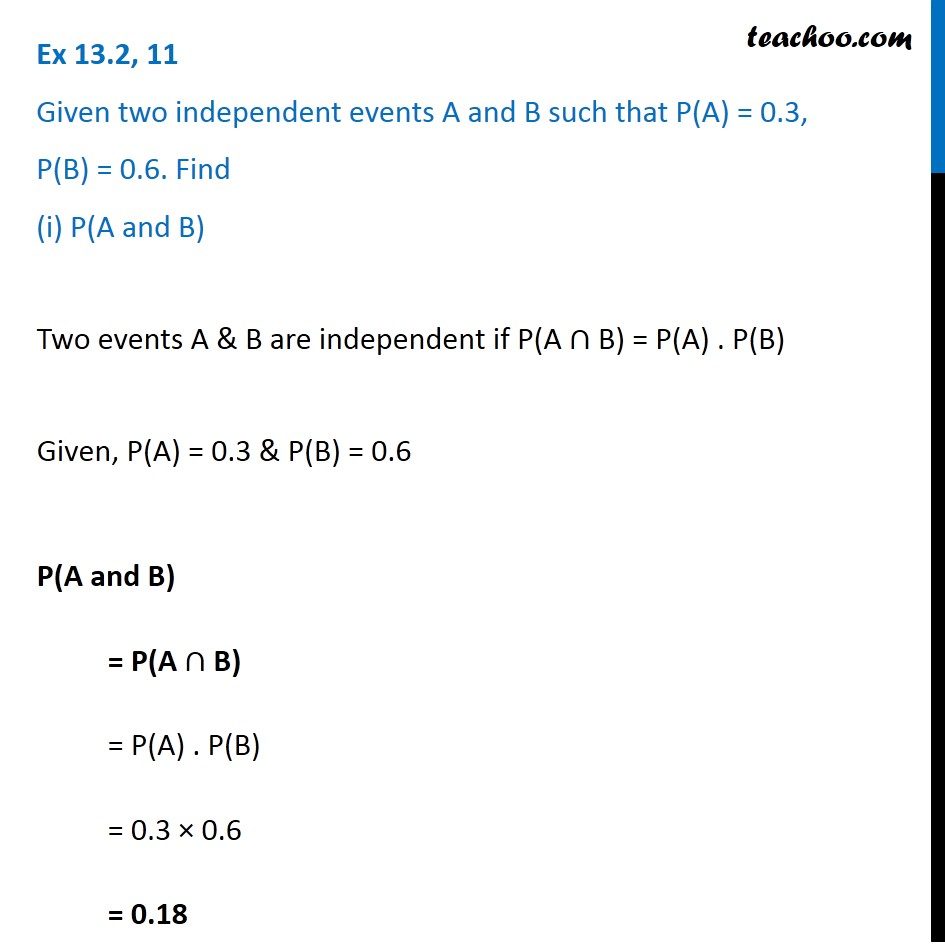Chapter 13 Class 12 Probability

Class 12
Important Questions for exams Class 12Learn in your speed, with individual attention - Teachoo Maths 1-on-1 Class

### Transcript

Ex 13.2, 11 Given two independent events A and B such that P(A) = 0.3, P(B) = 0.6. Find (i) P(A and B) Two events A & B are independent if P(A ∩ B) = P(A) . P(B) Given, P(A) = 0.3 & P(B) = 0.6 P(A and B) = P(A ∩ B) = P(A) . P(B) = 0.3 × 0.6 = 0.18